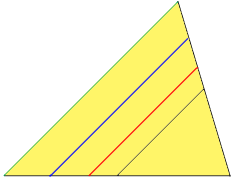SEARCH HOMEMath Central Quandaries & QueriesQuestion from Faith, a student: Does the measure of angle determine the length of its side? For example two angles are congruent then the sides are also congruent because from my understanding the angle determine the shape of triangle.Hi Faith,

The shape of a triangle is determined by the measures of its angles but the size of the triangle is not. The four triangles in the diagram below have corresponding angles congruent but the triangles of of different sizes. If the corresponding angles of two triangles are congruent then the triangles are said to be similar.I hope this helps,
PennyMath Central is supported by the University of Regina and the Imperial Oil Foundation.